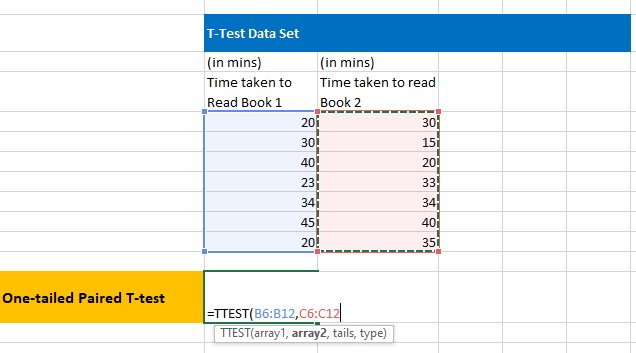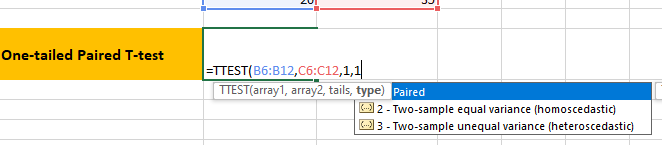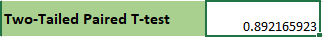### How To Test Statistical Significance By T-Test

In MS Excel, we can easily test statistical significance by using the function TTEST Let’s first understand the parameters of this function.

=TTEST(array1, array2, tails, type)

Array1 (Required): Range for dataset 1

Array2 (Required): Range for dataset 2

Tails (Required): This determines if it is a one-tail T-test or two tail T-test.

• One tail T-test
• Two tail T test

Type (required): Type of t-test

• Paired T-test, i.e. if the two data sets are related to each other
•  Unpaired T-test, the two samples have equal variance
•  Unpaired T-test , the two samples have unequal variance

Example:

1. Consider the following dataset for T-test.2. Select the two –datasets in array 1 and array 2.3. On an Empty Cell, write the formula to perform 1-tail Paired T testProbability obtained4. Similarly, perform a two–tailed paired T testProbability ObtainedBased on the obtained value you can accept or reject the null hypothesis. If the T-test gives the probability less than 0.05, then reject the null hypothesis. This means there is less than 5% probability that the null hypothesis is true.

Here, in both cases, p-value> 0.05. So, we accept the null hypothesis.

Errors

• #NUM! – If the arguments for tail or type are not correct. Tail argument must be 1 or 2. Type argument must be 1,2 or 3.
• #N/A! – The datasets are of different length
• #VALUE – The tail-argument or the type argument are non-numeric

## Template

```Further reading: